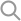PredictionIO's DASE architecture brings the separation-of-concerns design principle to predictive engine development. DASE stands for the following components of an engine:

• Data - includes Data Source and Data Preparator
• Algorithm(s)
• Serving
• Evaluator

Let's look at the code and see how you can customize the engine you built from the Vanilla Engine Template.

Evaluator will not be covered in this tutorial.

Before you use Vanilla template to develop your engine, it's recommended that you go through the DASE explanation of one of the other templates (e.g. Recommemdation template, Classification template) to see a concrete example of how the DASE components are used.

## Algorithm

PredictionIO supports two types of algorithms:

• P2LAlgorithm: trains a Model which does not contain RDD
• PAlgorithm: trains a Model which contains RDD

### P2LAlgorithm

By default, the Algorithm of the Vanilla template trains a simple model which does not contain RDD, as you can see in Algorithm.scala:

 1 2 3 4 5 class Model(val mc: Int) extends Serializable { override def toString = s"mc=${mc}" }  In this case, the Algorithm class extends P2LAlgorithm, as you can see in Algorithm.scala:  1 2 3 4 5 6 7 8 9 10 11 12 13 14 15 16 17 18 19 class Algorithm(val ap: AlgorithmParams) // extends PAlgorithm if Model contains RDD[] extends P2LAlgorithm[PreparedData, Model, Query, PredictedResult] { ... def train(sc: SparkContext, data: PreparedData): Model = { // Simply count number of events // and multiple it by the algorithm parameter // and store the number as model val count = data.events.count().toInt * ap.mult new Model(mc = count) } ... }  For P2LAlgorithm, the Model is automatically serialized and persisted by PredictionIO after training. You may also refer to Classification engine template for another example of P2LAlgorithm. ### PAlgorithm PAlgorithm should be used when your Model contains RDD. The model produced by PAlgorithm is not persisted by default. To persist the model, you need to do the following: • The Model class should extend the IPersistentModel trait and implement the save() method for saving the model. The trait IPersistentModel requires a type parameter which is the class type of algorithm parameter. • Implement a Model factory object which extends the IPersistentModelLoader trait and implement the apply() for loading the model. The trait IPersistentModelLoader requires two type parameters which are the types of algorithm parameter and the model produced by the algorithm. For example, let's say we add a new field mRdd which is type of RDD[Int] to the Vanilla template's Model class. The Model class is modified as following:  1 2 3 4 5 6 7 8 9 10 11 12 13 14 15 16 17 18 19 20 21 22 class Model( val mc: Int, val mRdd: RDD[Int] // ADDED ) extends IPersistentModel[AlgorithmParams] with Serializable { // ADDED // ADDED def save(id: String, params: AlgorithmParams, sc: SparkContext): Boolean = { sc.parallelize(Seq(mc)).saveAsObjectFile(s"/tmp/${id}/mc") mRdd.saveAsObjectFile(s"/tmp/${id}/mRdd") true } override def toString = { s"mc=${mc}" + // ADDED for debugging s"mRdd=[${mRdd.count()}] (${mRdd.take(2).toList}...)" } } 

Notice that it extends IPersistentModel[AlgorithmParams] and implement the save() method.

Next, we need to implement a Model factory object to load back the persisted model and return the Model instance:

 1 2 3 4 5 6 7 8 9 10 11 12 13 // ADDED object Model extends IPersistentModelLoader[AlgorithmParams, Model] { def apply(id: String, params: AlgorithmParams, sc: Option[SparkContext]) = { new Model( mc = sc.get.objectFile[Int](s"/tmp/${id}/mc").first, mRdd = sc.get.objectFile(s"/tmp/${id}/mRdd") ) } } 

At last, the Algorithm class needs to extend PAlgorithm and generate the RDD data for the new mRdd field in Model:

 1 2 3 4 5 6 7 8 9 10 11 12 13 14 15 16 17 18 19 20 21 22 23 24 25 26 27 28 class Algorithm(val ap: AlgorithmParams) extends PAlgorithm[PreparedData, Model, Query, PredictedResult] { // MODIFIED ... def train(sc: SparkContext, data: PreparedData): Model = { // Simply count number of events // and multiple it by the algorithm parameter // and store the number as model val count = data.events.count().toInt * ap.mult // ADDED // get the spark context val sc = data.events.context // create dummy RDD[Int] for demonstration purpose val mRdd = sc.parallelize(Seq(1,2,3)) new Model( mc = count, mRdd = mRdd // ADDED ) } ... } `

You may also refer to Similar Product engine template for another example of PAlgorithm.